# Solution assignment 11 Quadratic equations (factorizing)

### Assignment 11

Try to solve by factorizing: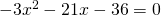### Solution

In this equation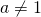, but both sides of the equation can be divided by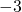, giving the equation:Next we have to find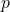and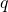such that: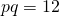and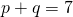The following values will do: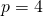and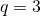Thus the equation can be written as: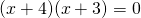and thus the solutions are: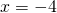or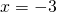0